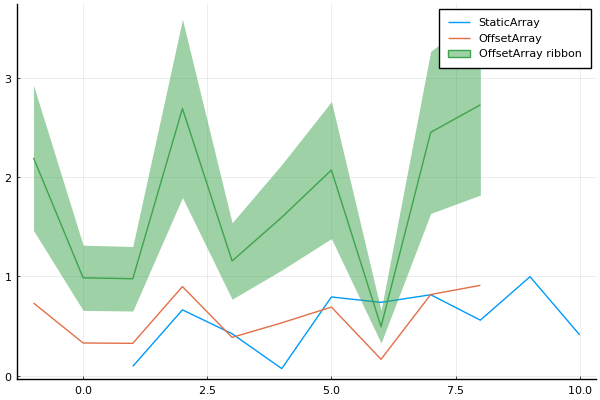# Array Typesusing Plots
pyplot()

Plots supports different Array types that follow the AbstractArray interface, like StaticArrays and OffsetArrays.

begin
using StaticArrays, OffsetArrays
sv = SVector{10}(rand(10))
ov = OffsetVector(rand(10), -2)
plot([sv, ov], label = ["StaticArray" "OffsetArray"])
plot!(3ov, ribbon = ov, label = "OffsetArray ribbon")
end